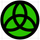cancel
Showing results for
Did you mean:
cancel
Showing results for
Did you mean:

Got a minute? We appreciate your feedback:

GDL
About building parametric objects with GDL.

## values{2} List - Custom Parameter QuestionEnthusiast

Hi,

I have a values{2} list. The list entries are standard lengths of timber. I would like the last entry as Custom, to enable the user to enter a value for variable Timb_L i.e.,

values{2} "Timb_L",  0, ` Length A:`,

1, `Length B:`,

2, ` Custom:`

My hope was to use “” as the result for the user selecting #2 i.e.,

IF Timb_L = 0    THEN Timb_L = 1000

IF Timb_L = 1    THEN Timb_L = 1050

IF Timb_L = 2    THEN Timb_L = “”

PARAMETER    Timb_L = Timb_L

However when I add this “” part of the code into a larger script, the UI keeps blanking out as soon as item 2 is selected. Is there a way to provide an IF THEN which allows the user to manually enter a value using values{2}?

I also tried:

IF Timb_L = 2    THEN Timb_L = Timb_L

PARAMETER    Timb_L = Timb_L

And I tried not even having a value for 2:

IF Timb_L = 0    THEN Timb_L = 1000

IF Timb_L = 1    THEN Timb_L = 1050

PARAMETER    Timb_L = Timb_L

Many thanks, Matt

1 ACCEPTED SOLUTION
2 REPLIES 2
SolutionLegend

You need two parameters TL1 & TL2.

Your object uses the value in TL2

If TL1  = 0 then TL2 = 1000 and lock or hide TL2

If TL1  = 1 then TL2 = 1050 and lock or hide TL2

If TL1 =2 then do nothing but unlock TL2 for manual entry

You may want to set a range of values for TL2 to control custom entry or preset lengths.

Apple iMac macOS Sonoma / AC27UKI (most recent builds)Enthusiast

Oh that is brilliant, thank you so much DGSketcher!Latest solutions

Most liked posts